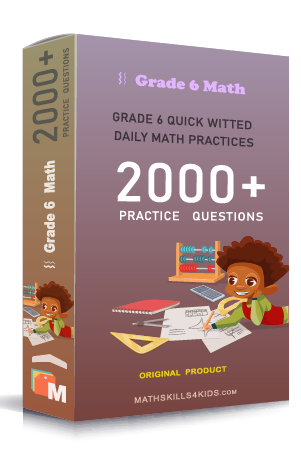# Grade 6 Dividing fraction worksheets with solutions

Subject
Math

Resource Type
Worksheets, Printables, Homeschool

Standards
CCSS 6.NS.A.1

Format
PDF (11.3 MB | 16 pages → Exercises + Solutions)

• ### -15% OFF Over \$100

Promo Code: TOPLA

After Purchase

\$2.15

This package allows you to practice the following skills

Dividing whole numbers by unit fractions using models

Finding the reciprocal of fraction or whole number

Dividing whole numbers and unit fractions

Solving the division of fractions by whole numbers in recipes

Dividing two fractions

Estimating quotients when dividing mixed numbers

Dividing fractions and mixed numbers

Dividing fractions and mixed numbers word problems

This product is a part of the Mega Pack
* Grade 6 quick-witted daily math practices *